Thermodynamics and Propulsion

6.2 The Thermodynamic Temperature Scale

The considerations of Carnot cycles in this section have not mentioned the working medium. They are thus not limited to an ideal gas and hold for Carnot cycles with any medium. Earlier we derived the Carnot efficiency with an ideal gas as a medium and the temperature definition used in the ideal gas equation was not essential to the thermodynamic arguments. More specifically, we can define a thermodynamic temperature scale that is independent of the working medium. To see this, consider the situation shown below in Figure 6.2, which has three reversible cycles. There is a high temperature heat reservoir at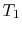and a low temperature heat reservoir at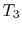. For any two temperatures,, the ratio of the magnitudes of the heat absorbed and rejected in a Carnot cycle has the same value for all systems.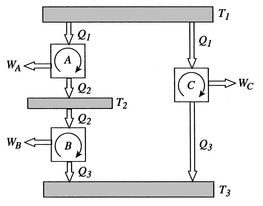We choose the cycles so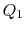is the same for A and C. Also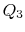is the same for B and C. For a Carnot cycleAlso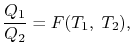But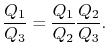HenceWe thus conclude that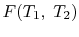has the form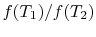, and similarly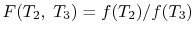. The ratio of the heat exchanged is thereforeIn general,so that the ratio of the heat exchanged is a function of the temperature. We could choose any function that is monotonic, and one choice is the simplest: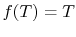. This is the thermodynamic scale of temperature,. The temperature defined in this manner is the same as that for the ideal gas; the thermodynamic temperature scale and the ideal gas scale are equivalent.

Douglas Quattrochi 2006-08-06